# How to make an Arduino robot | Low cost Arduino Robot Tutorial

## How to make an Arduino Robot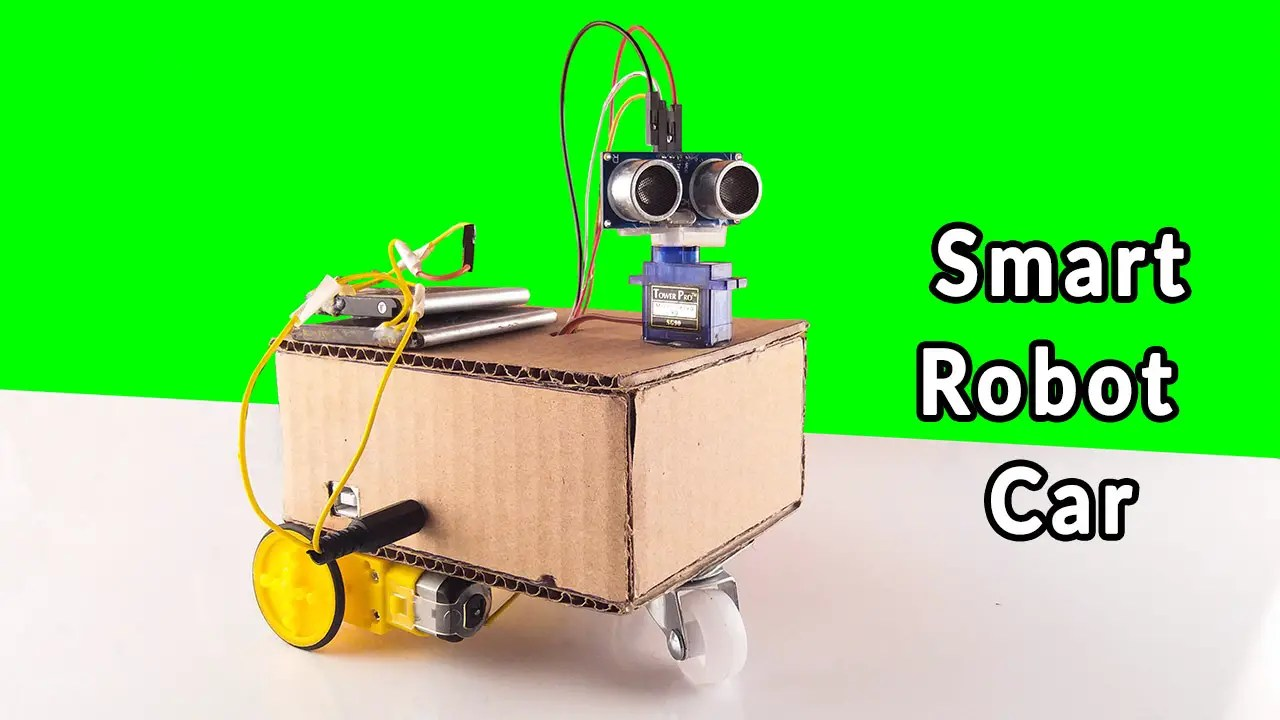Hello guys, welcome back to my SriTu Hobby. Through this tutorial, we will talk about how to make an Arduino robot. Also, this Arduino robot tutorial is presented step by step so that anyone can understand. It mainly uses Arduino UNO board, l298n motor driver board, ultrasonic sensor, and servo motor. We have presented step by step how to build a robot using Arduino in two previous articles. Click on the links below to read those articles.

Tutorial 1 — Read me

Tutorial 2 — Read me

### Arduino Robot Process

This robot works in two main ways. For this, two codes are included in the same program.

#### 1.Obstacle avoidance robot.

Here the ultrasonic sensor calculates the forward distance as the Arduino robot moves forward. This Arduino robot will stop if the distance between the obstacle and the Arduino robot is less than 9cm when it encounters an obstacle in front. Afterward, the servo motor then turns the ultrasonic sensor to either side. At that point, the ultrasonic sensor calculates the distances on either side. Then, using that distance, the program finds the longest side without an obstacle by a simple equation. After, the Arduino robot turns to that side and moves forward again. This process continues.

#### 2.Cute Robot

When the hand is close to the ultrasonic sensor, the robot moves forward and backward twice and shakes the ultrasonic sensor to either side. This is done by the servo motor.

### Step 1

Firstly, identify these components.

#### Arduino UNO board.#### Phone battery.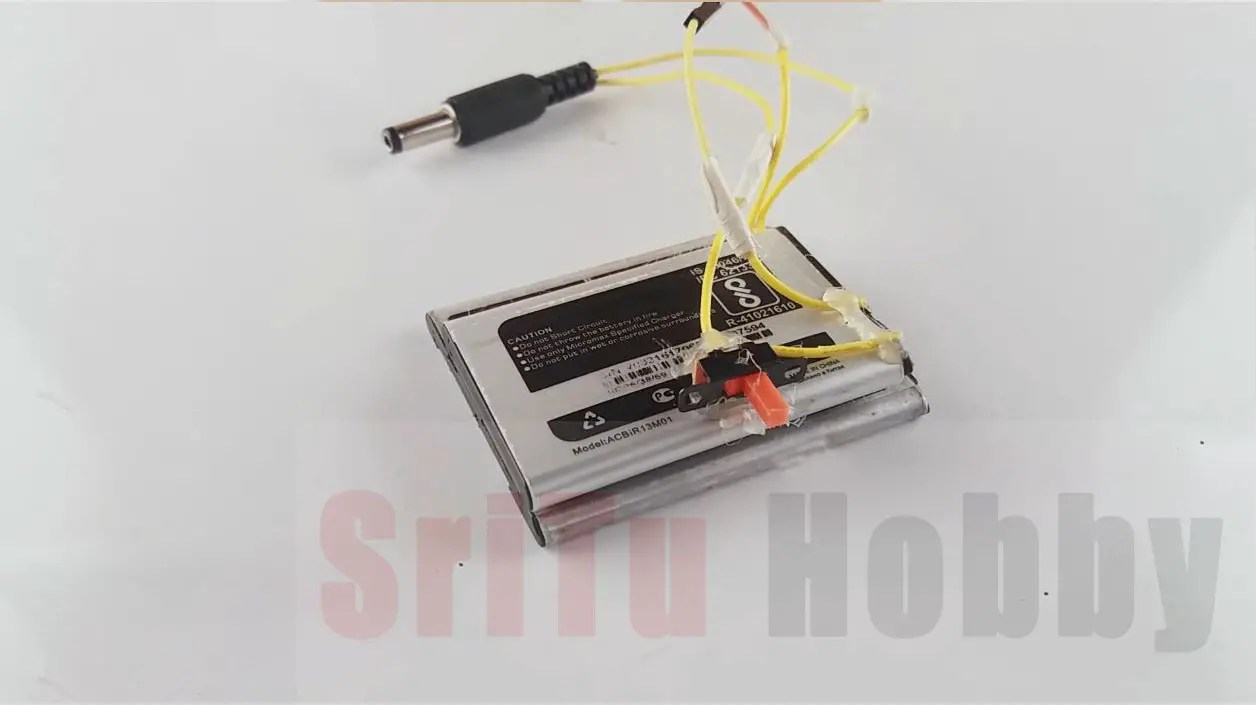### Step 2

Secondly, cut a 5 * 4-inch piece of cardboard. Then connect the two gear motors as shown below. Use a Glue gun to connect the gear motor.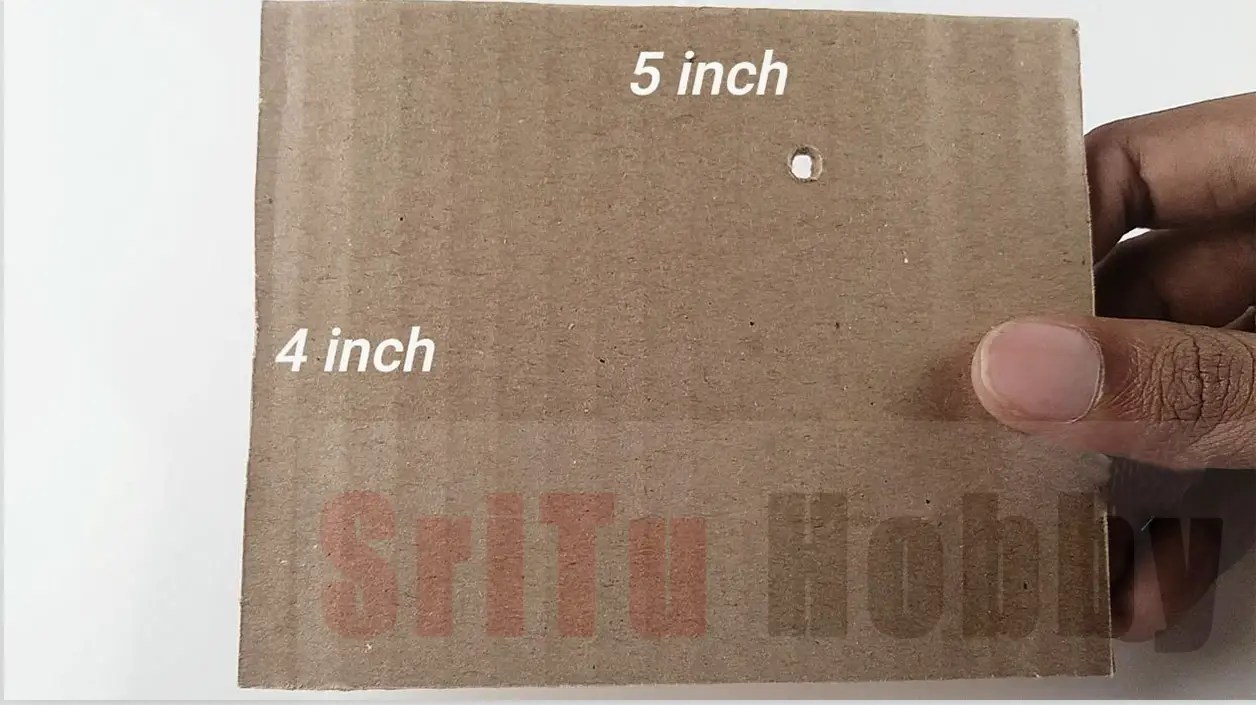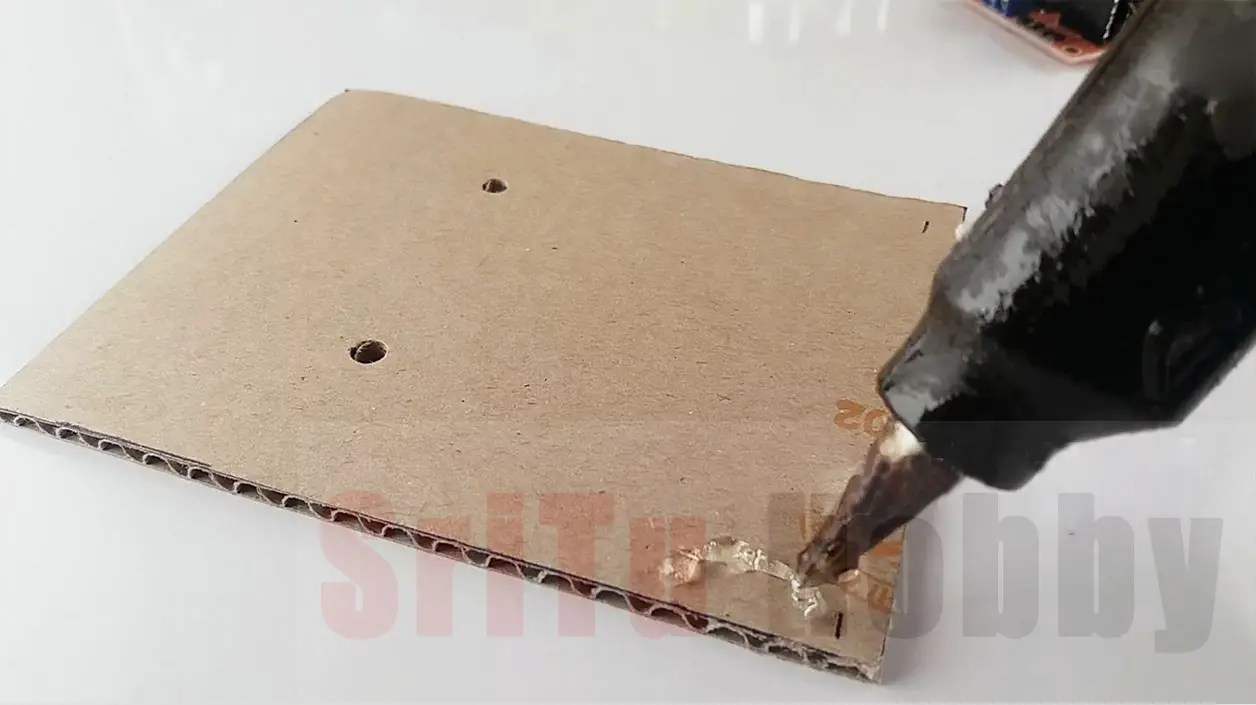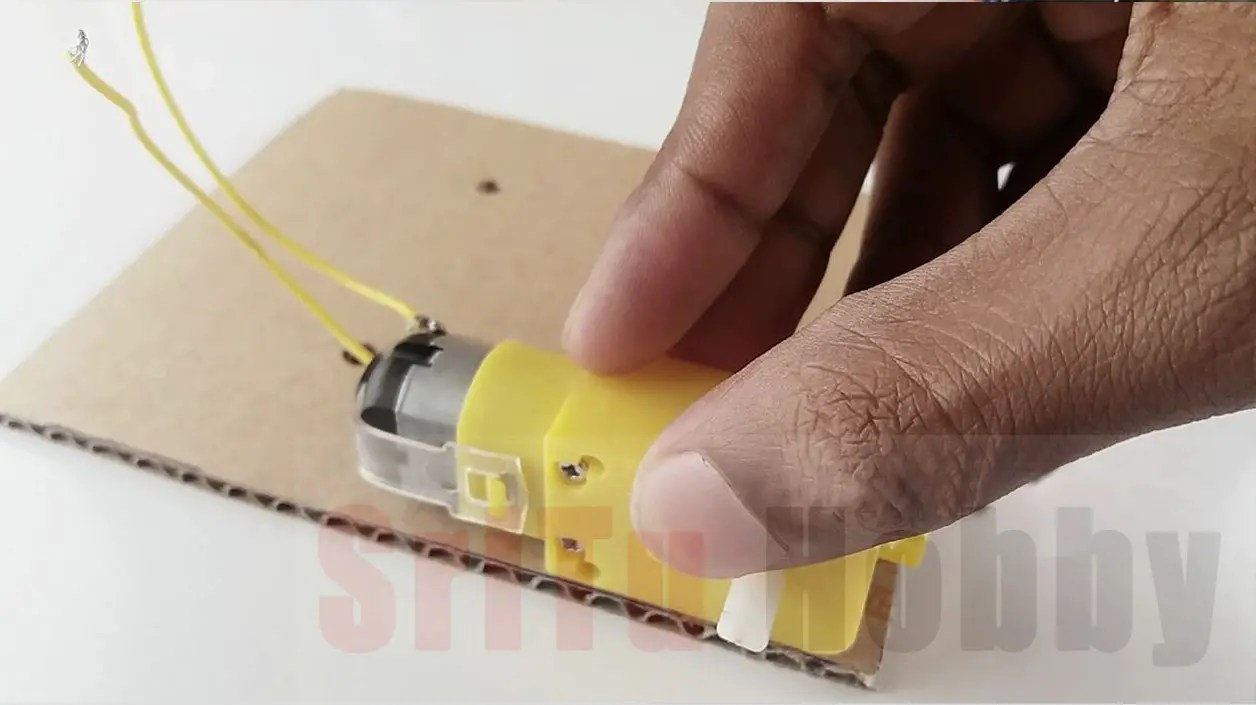### Step 3

Thirdly, attach the single robot wheel to the piece of cardboard as shown below.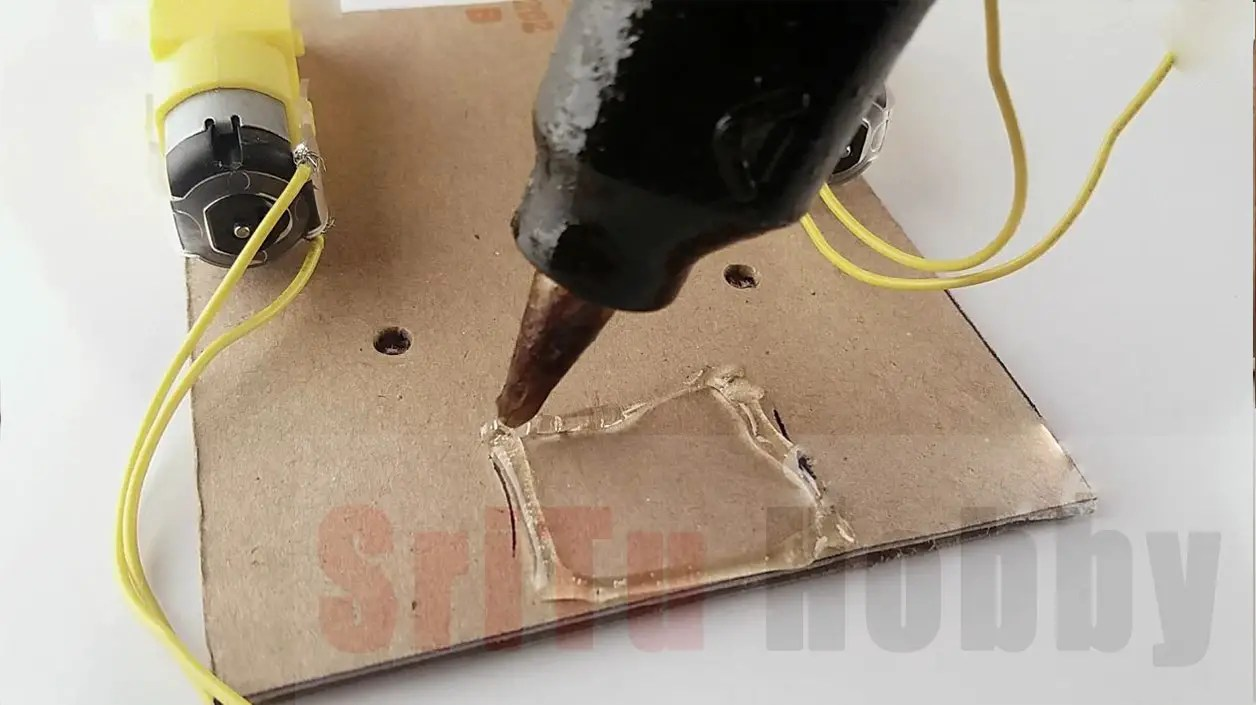### Step 4

Then attach the motor driver board to the top of the cardboard and connect the two gear motors to it. Use the circuit diagram below.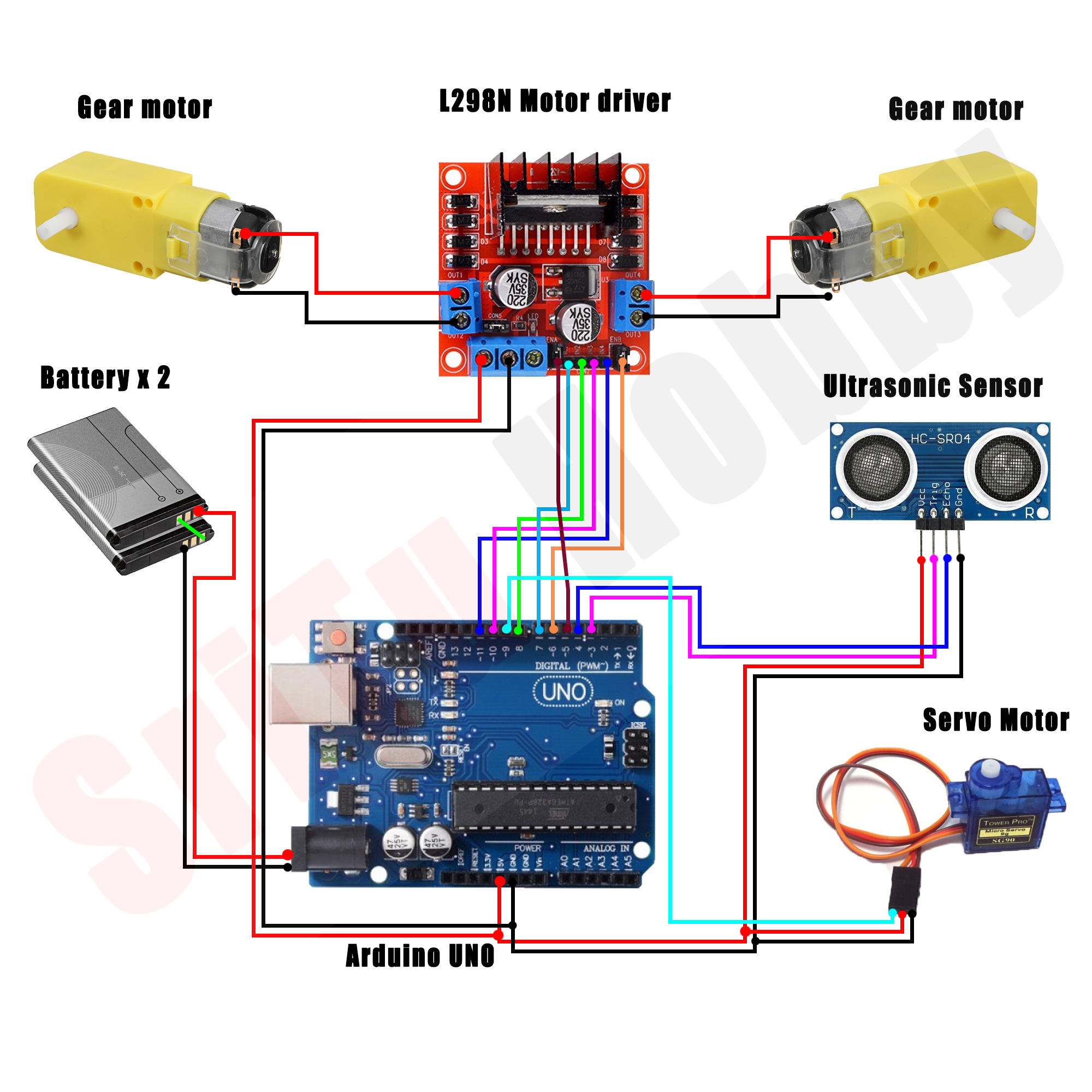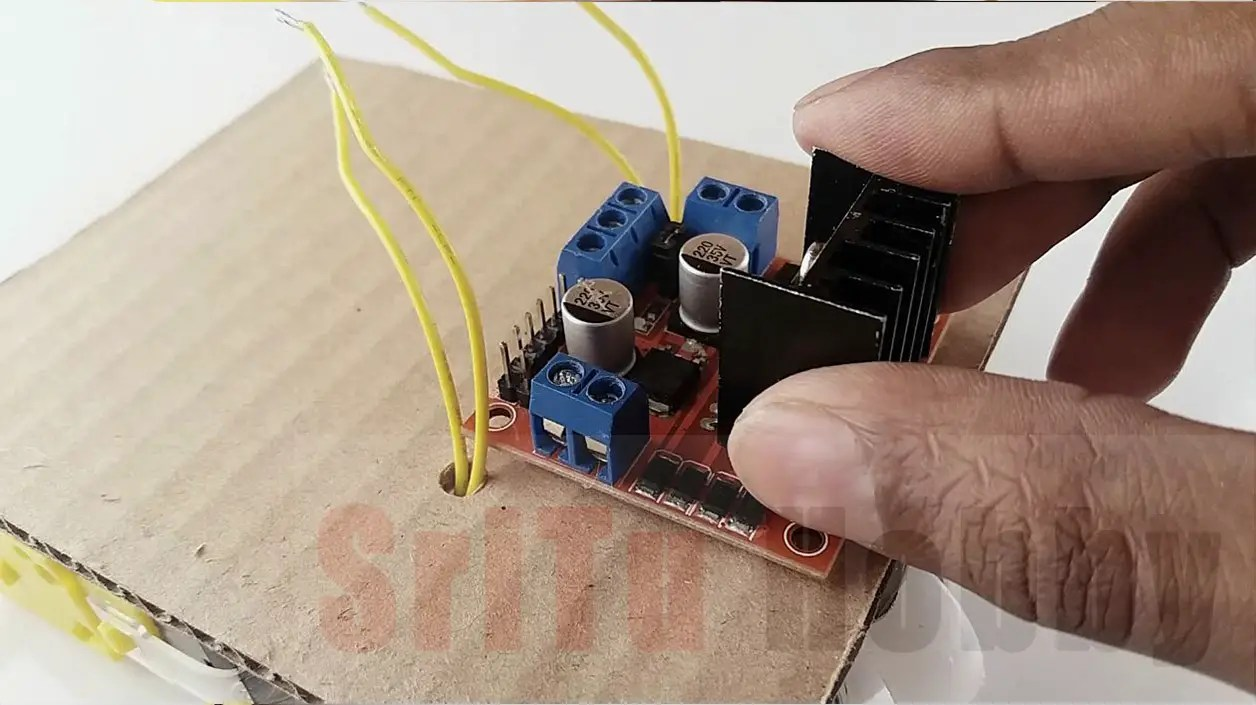### Step 5

Next, cut two pieces of cardboard measuring 5 * 2 inches in size. Then glue that piece to the main cardboard piece.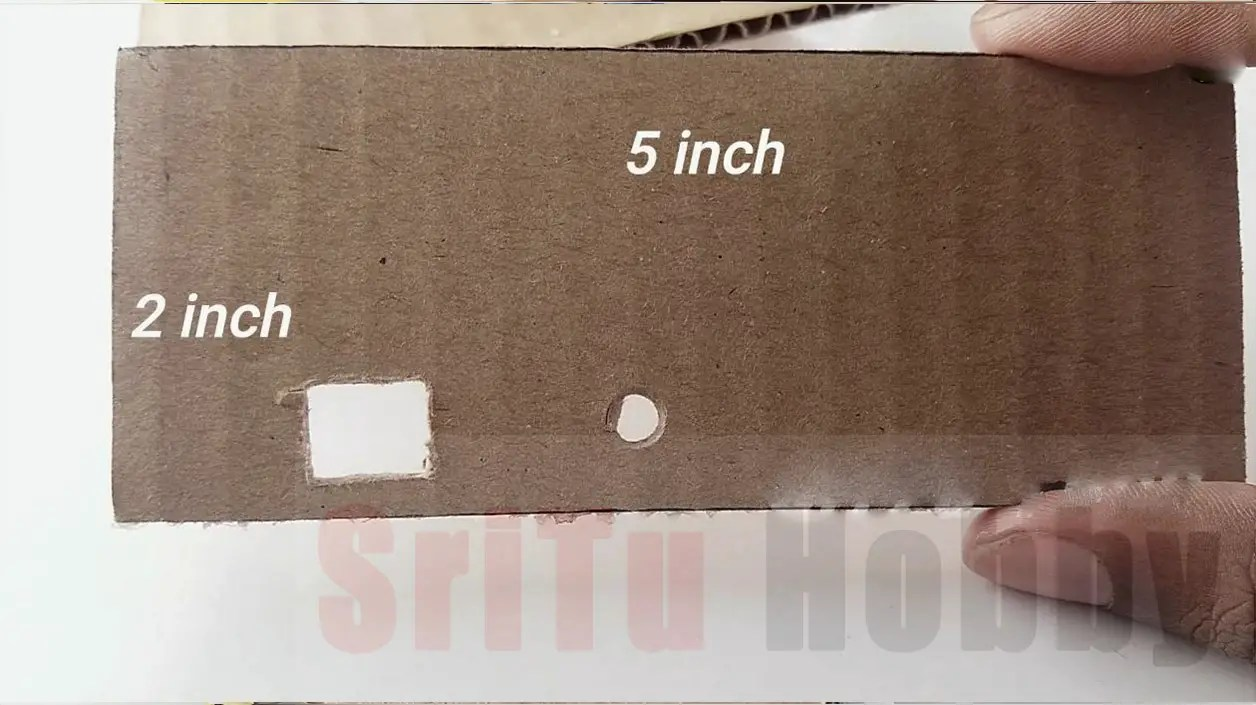### Step 6

Afterward, connect the Arduino board as shown below.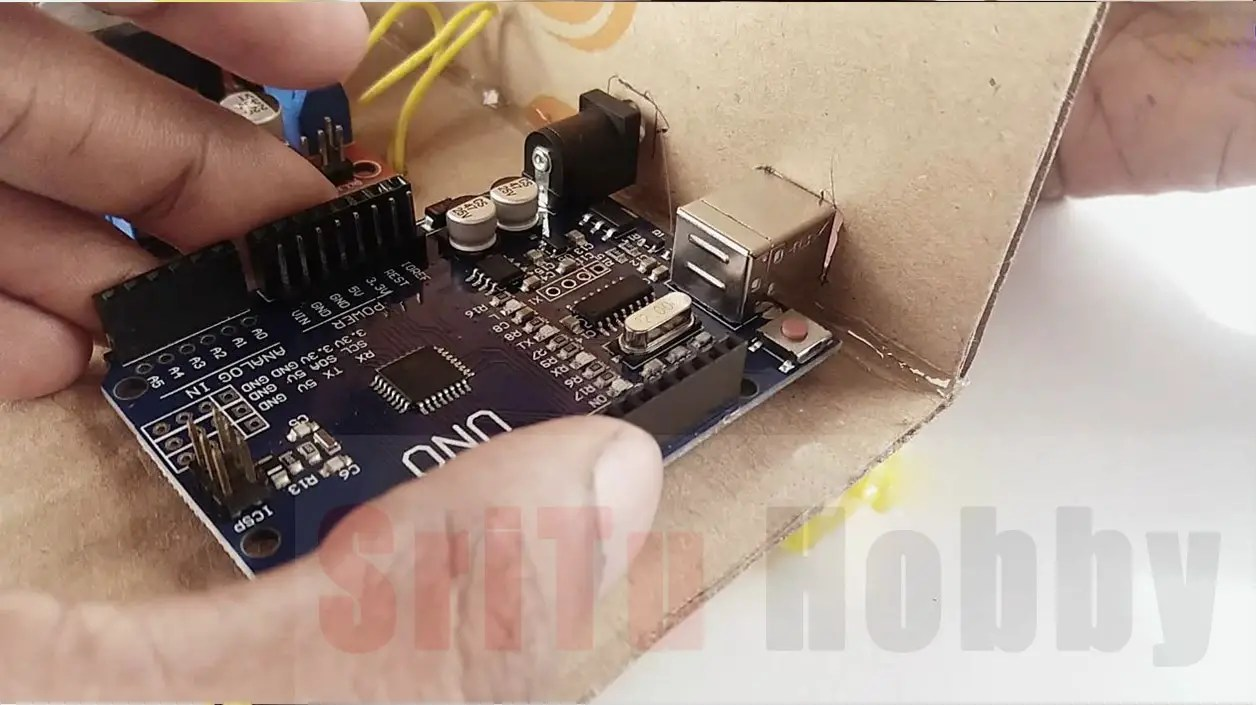### Step 7

Now, cut a 5 * 4-inch piece of cardboard again and connect the servo motor to it. Then connect the ultrasonic sensor to the top of the servo motor.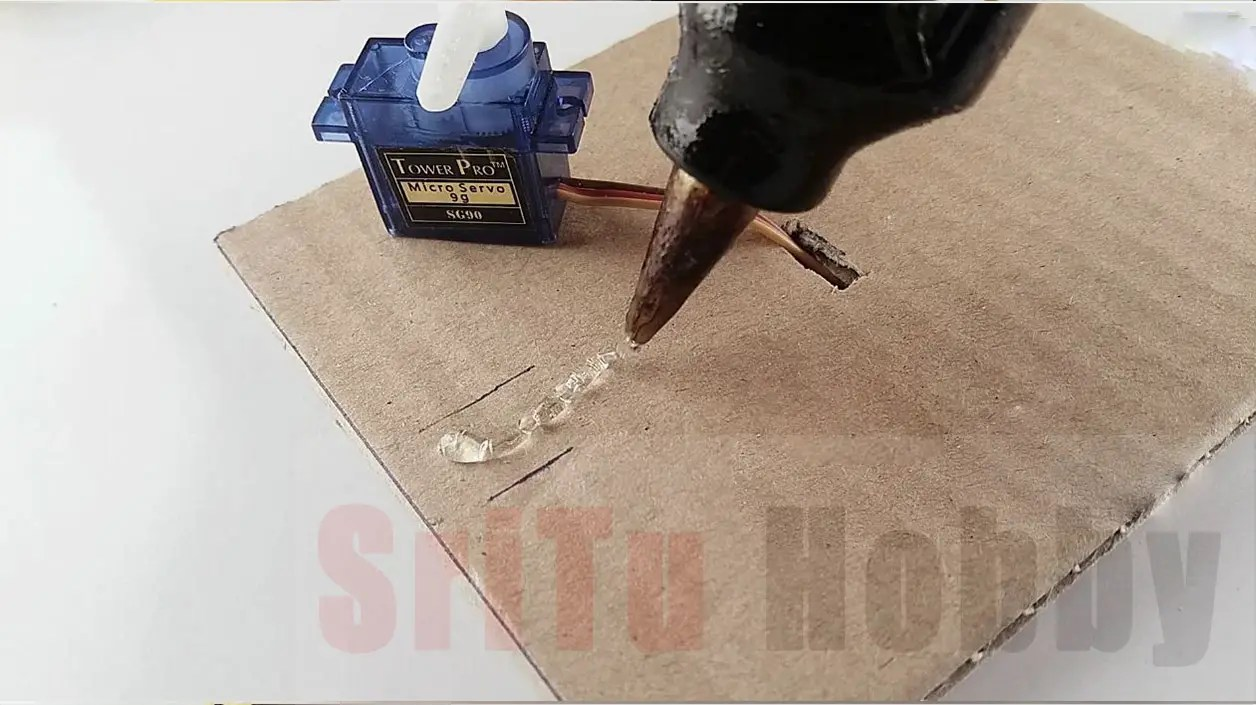### Step 8

Next, connect the ultrasonic sensor and servo motor to the Arduino board. Use the circuit diagram above for that.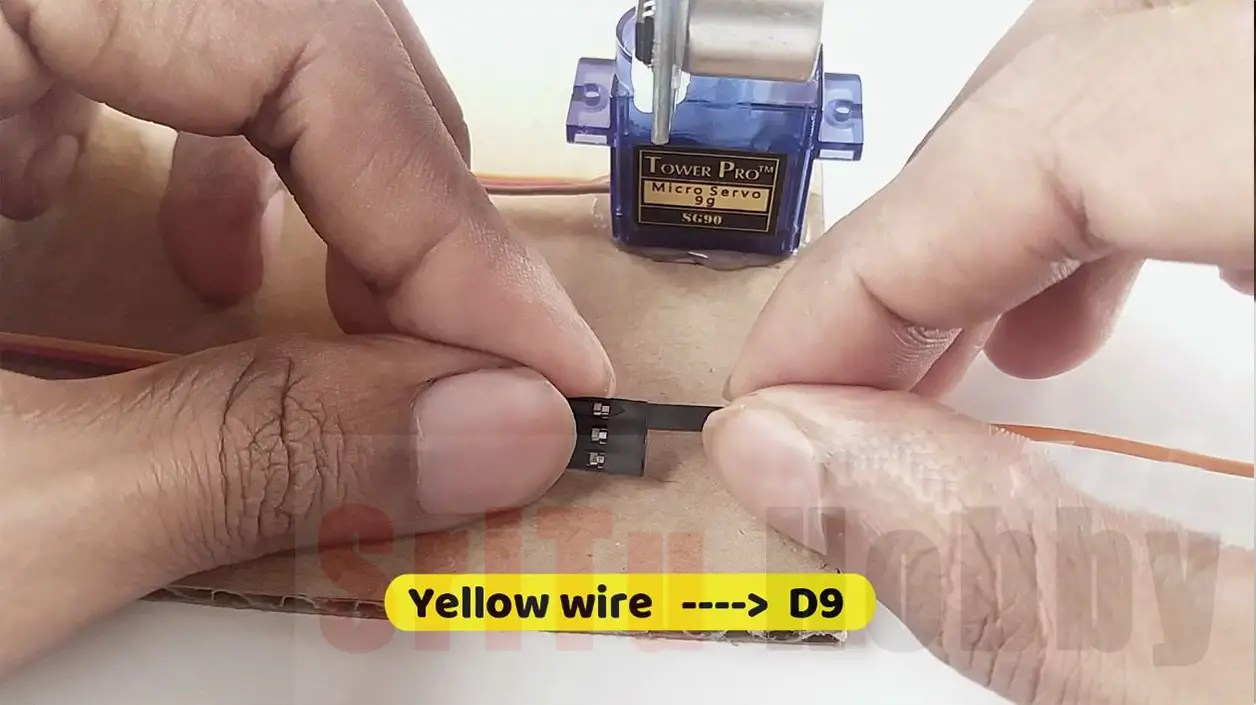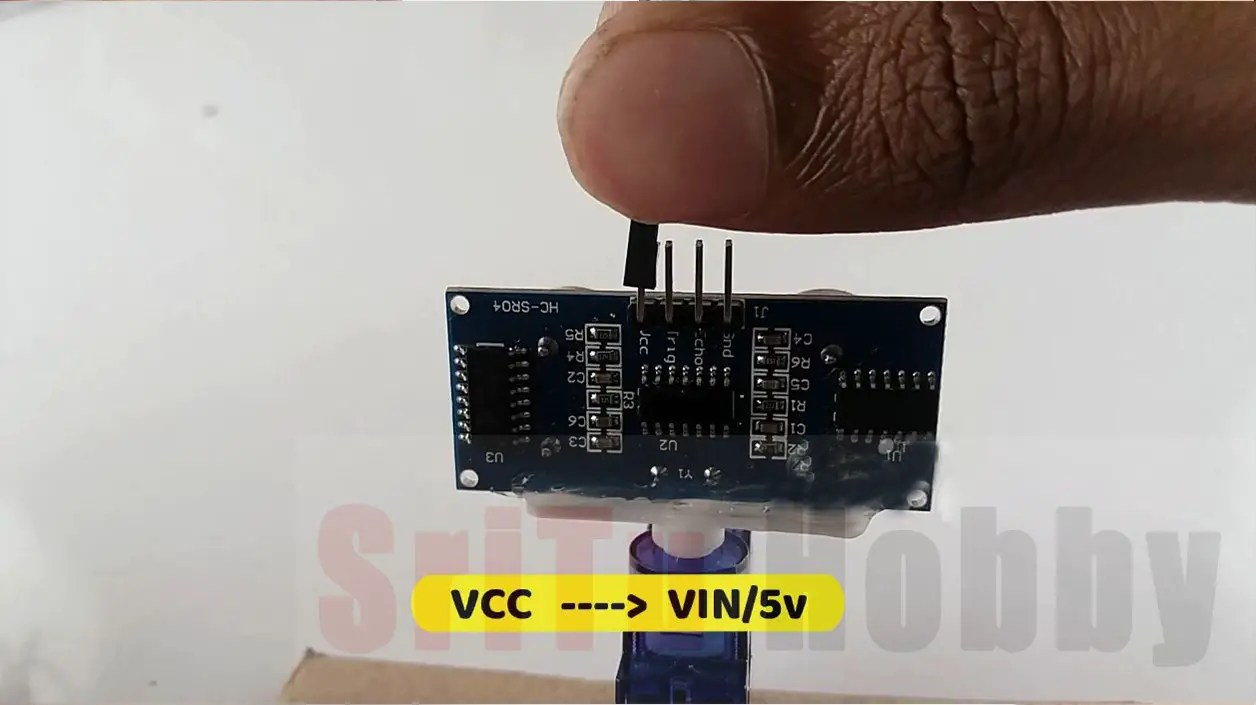### Step 9

Now, cut two pieces of cardboard measuring 4 * 2 in size and paste them as shown below. Also, glue the piece of cardboard of size 5 * 2 that we cut earlier.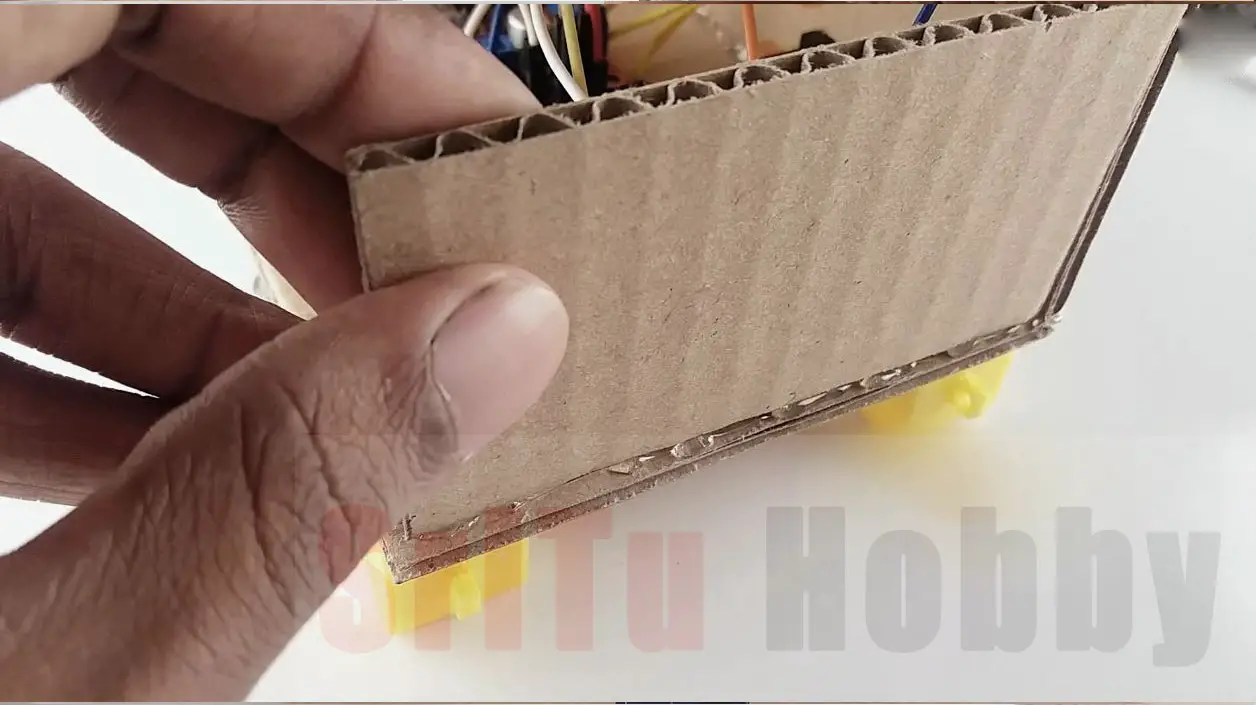### Step 10

Then connect the motor driver board to the Arduino board. Afterward, connect the gear wheels for the gear motors.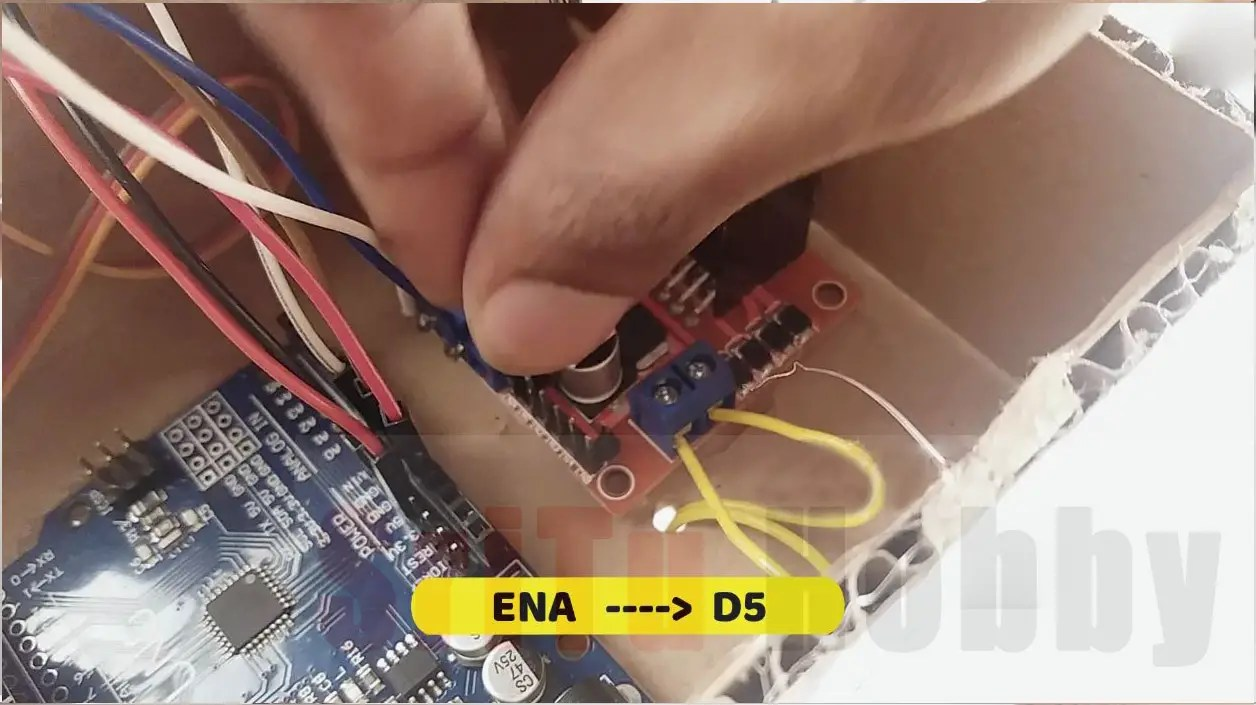### Step 11

Well, now we will create the required program for this Arduino robot. Firstly, connect the Arduino robot to the computer. The required program for this is given below.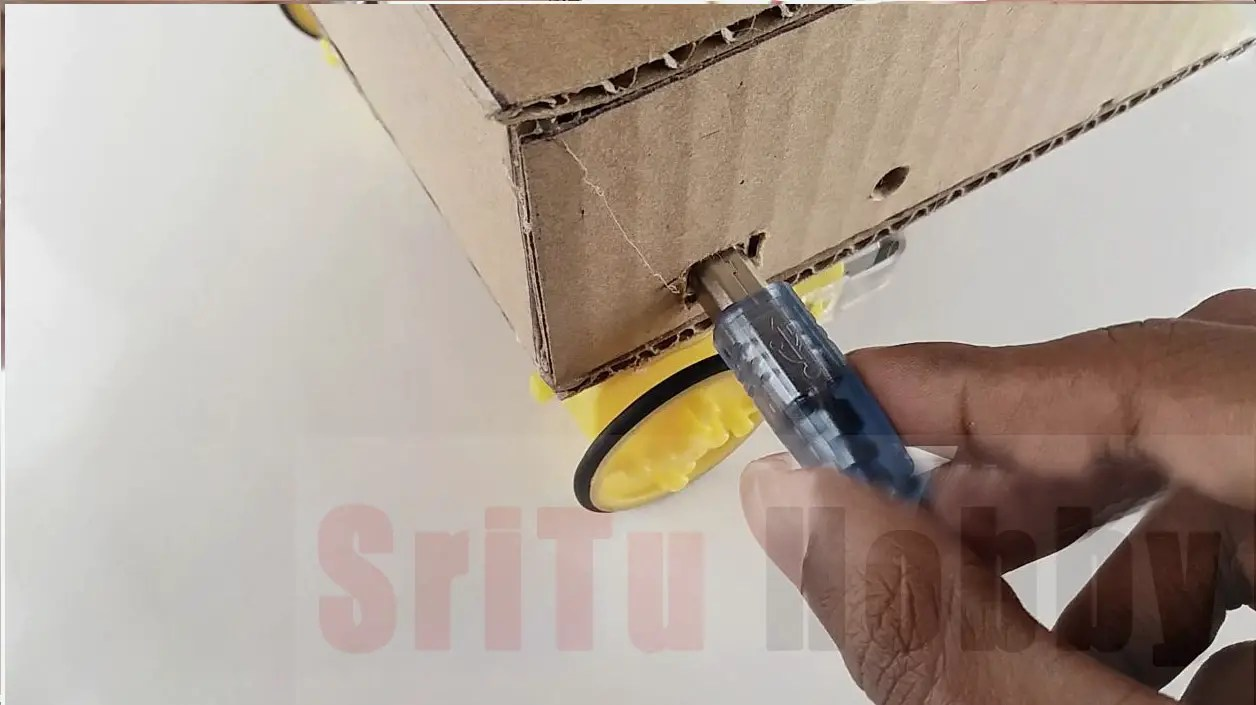The complete program of this project – Download
``````/*Arduino Robot.
created by the SriTu Tech team.
Read the code below and use it for any of your creations.
*/

#include <Servo.h>//include servo library
Servo servo;//create object
int Spoint = 105; //servo center point
int Lvalue;
int Rvalue;
int dis;
bool change;

//motor 0ne
#define ENA 5
#define IN1 7
#define IN2 8

//motor two
#define ENB 6
#define IN3 10
#define IN4 11

void setup() {
Serial.begin(9600);//enable serial monitor
pinMode(3, OUTPUT);//trig pin
pinMode(4, INPUT);//echo pin
pinMode(ENA, OUTPUT);//motor pins
pinMode(ENB, OUTPUT);//motor pins
pinMode(IN1, OUTPUT);//motor pins
pinMode(IN2, OUTPUT);//motor pins
pinMode(IN3, OUTPUT);//motor pins
pinMode(IN4, OUTPUT);//motor pins
servo.attach(9);//define servo pin
servo.write(Spoint);//servo motor start point
delay(2000);
start();
}

void loop() {
cuterobot();//following code
//obstacle ();//obstacle code
}

void obstacle () {
dis = distance ();
if (dis <= 9) {
motors('S', 0);
motors('B', 250);
delay(50);
motors('S', 0);
Lvalue = leftsee();
Serial.println(Lvalue);
Rvalue = rightsee();
Serial.println(Rvalue);
if (Lvalue < Rvalue) {
motors('R', 130);
delay(300);
motors('S', 0);
} else if (Lvalue > Rvalue) {
motors('L', 130);
delay(300);
motors('S', 0);
}
} else {
motors('F', 130);
}

}

void cuterobot() {
byte stay = 0;
change = true;

dis = distance ();
if (dis <= 7 ) {
motors('B', 220);
delay(250);
motors('S', 0);
for (byte a = 0; a < 4; a++) {
servo.write(90);
delay(100);
servo.write(120);
delay(100);
servo.write(Spoint);
}
while (change) {
motors('F', 220);
delay(150);
motors('B', 220);
delay(150);
motors('S', 0);
delay(2000);
stay++;
Serial.println("updown");
if (stay == 3) {
for (byte a = 0; a < 4; a++) {
servo.write(90);
delay(100);
servo.write(120);
delay(100);
servo.write(Spoint);
}
change = false;
Serial.println("rotete");
}
}

} else if (dis >= 10 && dis <= 15) {
motors('F', 200);
change = true;
Serial.println("turn");
} else {
motors('S', 0);
}
}

int distance () {
digitalWrite(3, LOW);
delayMicroseconds(4);
digitalWrite(3, HIGH);
delayMicroseconds(10);
digitalWrite(3, LOW);

int t = pulseIn(4, HIGH);//input pulse and save it veriable
int cm = t / 29 / 2; //time convert distance
return cm;
}

//backward ='B',Forward ='F',turn left ='L',turn right ='R',stop ='S'
void motors(char action, int Speed) {
if (action == 'B') {
digitalWrite(ENA, Speed);
digitalWrite(ENB, Speed);
digitalWrite(IN1, HIGH);
digitalWrite(IN2, LOW);
digitalWrite(IN3, LOW);
digitalWrite(IN4, HIGH);
} else if (action == 'F') {
digitalWrite(ENA, Speed);
digitalWrite(ENB, Speed);
digitalWrite(IN1, LOW);
digitalWrite(IN2, HIGH);
digitalWrite(IN3, HIGH);
digitalWrite(IN4, LOW);
} else if (action == 'L') {
digitalWrite(ENA, Speed);
digitalWrite(ENB, Speed);
digitalWrite(IN1, HIGH);
digitalWrite(IN2, LOW);
digitalWrite(IN3, HIGH);
digitalWrite(IN4, LOW);
} else if (action == 'R') {
digitalWrite(ENA, Speed);
digitalWrite(ENB, Speed);
digitalWrite(IN1, LOW);
digitalWrite(IN2, HIGH);
digitalWrite(IN3, LOW);
digitalWrite(IN4, HIGH);
} else if (action == 'S') {
digitalWrite(ENA, Speed);
digitalWrite(ENB, Speed);
digitalWrite(IN1, LOW);
digitalWrite(IN2, LOW);
digitalWrite(IN3, LOW);
digitalWrite(IN4, LOW);
}
}
void start() {
servo.write(0);
delay(500);
servo.write(180);
delay(500);
for (byte a = 0; a < 5; a++) {
servo.write(90);
delay(100);
servo.write(120);
delay(100);
}
servo.write(Spoint);
delay(200);
motors('L', 120);
delay(1000);
motors('S', 0);
}

int leftsee() {
servo.write(180);
dis = distance ();
delay(1000);
return dis;

}
int rightsee() {
servo.write(30);
dis = distance ();
delay(1000);
servo.write(Spoint);
return dis;
}``````

#### Code explanation

The Servo library is included here first. After, an object called “servo” is created for that.
#include <Servo.h>//include servo library
Servo servo;//create an object
Secondly, several variables have been created to support this program.
int Spoint = 105; //servo center point
int Lvalue;
int Rvalue;
int dis;
bool change;

Thirdly, the motor drive board connected PINs are defined.
//motor 0ne
#define ENA 5
#define IN1 7
#define IN2 8

//motor two
#define ENB 6
#define IN3 10
#define IN4 11
Then, the pins connecting the ultrasonic sensor, motor driver board, and servo motor in the Void setup are set to OUTPUT and INPUT PIN. Also, the number of degrees that the servo motor should have when operating the ARDUINO ROBOT is set. After, a function called “start” is included. This is described below.
void setup() {
Serial.begin(9600);//enable serial monitor
pinMode(3, OUTPUT);//trig pin
pinMode(4, INPUT);//echo pin
pinMode(ENA, OUTPUT);//motor pins
pinMode(ENB, OUTPUT);//motor pins
pinMode(IN1, OUTPUT);//motor pins
pinMode(IN2, OUTPUT);//motor pins
pinMode(IN3, OUTPUT);//motor pins
pinMode(IN4, OUTPUT);//motor pins
servo.attach(9);//define servo pin
servo.write(Spoint);//servo motor start point
delay(2000);
start();
}

The void loop contains two main functions. We can run these two functions one by one. For that, we need to comment on the function we do not need and after, upload the program. (Use two forward slash to comment //)
void loop() {
cuterobot();//following code
//obstacle ();//obstacle code
}
void obstacle ()  —> This includes the code used to identify an Arduino robot obstacle.
void obstacle () {
dis = distance ();
if (dis <= 9) {
motors(‘S’, 0);
motors(‘B’, 250);
delay(50);
motors(‘S’, 0);
Lvalue = leftsee();
Serial.println(Lvalue);
Rvalue = rightsee();
Serial.println(Rvalue);
if (Lvalue < Rvalue) {
motors(‘R’, 130);
delay(300);
motors(‘S’, 0);
} else if (Lvalue > Rvalue) {
motors(‘L’, 130);
delay(300);
motors(‘S’, 0);
}
} else {
motors(‘F’, 130);
}

}
void cuterobot()  —> This includes the code related to how an Arduino robot behaves.
void cuterobot() {
byte stay = 0;
change = true;

dis = distance ();
if (dis <= 7 ) {
motors(‘B’, 220);
delay(250);
motors(‘S’, 0);
for (byte a = 0; a < 4; a++) {
servo.write(90);
delay(100);
servo.write(120);
delay(100);
servo.write(Spoint);
}
int distance ()  —>  In this function, the distance is measure by the ultrasonic sensor and then the value is “return”.
int distance () {
digitalWrite(3, LOW);
delayMicroseconds(4);
digitalWrite(3, HIGH);
delayMicroseconds(10);
digitalWrite(3, LOW);

int t = pulseIn(4, HIGH);//input pulse and save it veriable
int cm = t / 29 / 2; //time convert distance
return cm;

}

void motors(char action, int Speed)  —>  This function includes forward, backward, left, right, and stop codes of the gear motor.
void motors(char action, int Speed) {
if (action == ‘B’) {
digitalWrite(ENA, Speed);
digitalWrite(ENB, Speed);
digitalWrite(IN1, HIGH);
digitalWrite(IN2, LOW);
digitalWrite(IN3, LOW);
digitalWrite(IN4, HIGH);
} else if (action == ‘F’) {
digitalWrite(ENA, Speed);
digitalWrite(ENB, Speed);
digitalWrite(IN1, LOW);
digitalWrite(IN2, HIGH);
digitalWrite(IN3, HIGH);
digitalWrite(IN4, LOW);
} else if (action == ‘L’) {
digitalWrite(ENA, Speed);
digitalWrite(ENB, Speed);
digitalWrite(IN1, HIGH);
digitalWrite(IN2, LOW);
digitalWrite(IN3, HIGH);
digitalWrite(IN4, LOW);
} else if (action == ‘R’) {
digitalWrite(ENA, Speed);
digitalWrite(ENB, Speed);
digitalWrite(IN1, LOW);
digitalWrite(IN2, HIGH);
digitalWrite(IN3, LOW);
digitalWrite(IN4, HIGH);
} else if (action == ‘S’) {
digitalWrite(ENA, Speed);
digitalWrite(ENB, Speed);
digitalWrite(IN1, LOW);
digitalWrite(IN2, LOW);
digitalWrite(IN3, LOW);
digitalWrite(IN4, LOW);
}
}
void start()   —>  Here is how the Arduino robot behaves when powered on.
void start() {
servo.write(0);
delay(500);
servo.write(180);
delay(500);
for (byte a = 0; a < 5; a++) {
servo.write(90);
delay(100);
servo.write(120);
delay(100);
}
servo.write(Spoint);
delay(200);
motors(‘L’, 120);
delay(1000);
motors(‘S’, 0);
}

int leftsee() —>  The servo motor turns to the left.
int leftsee() {
servo.write(180);
dis = distance ();
delay(1000);
return dis;
}
int rightsee()  —> The servo motor turns to the right
int rightsee() {
servo.write(30);
dis = distance ();
delay(1000);
servo.write(Spoint);
return dis;
}

### Step 12

So, now select board and port. Afterward, upload this code to the Arduino board. (Remember to select the function you want and upload it. The void loop includes two main functions. Comment on the unwanted function.)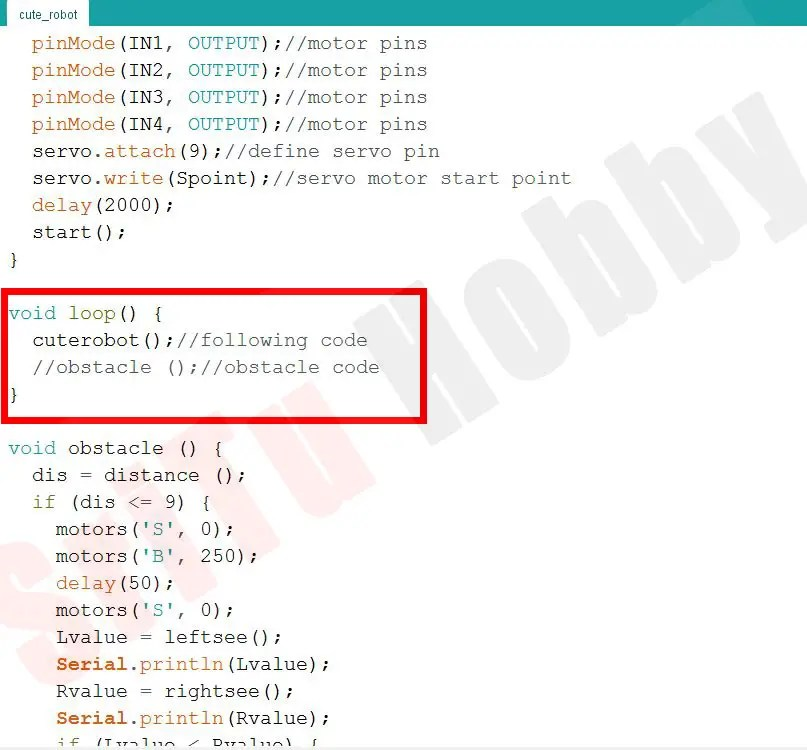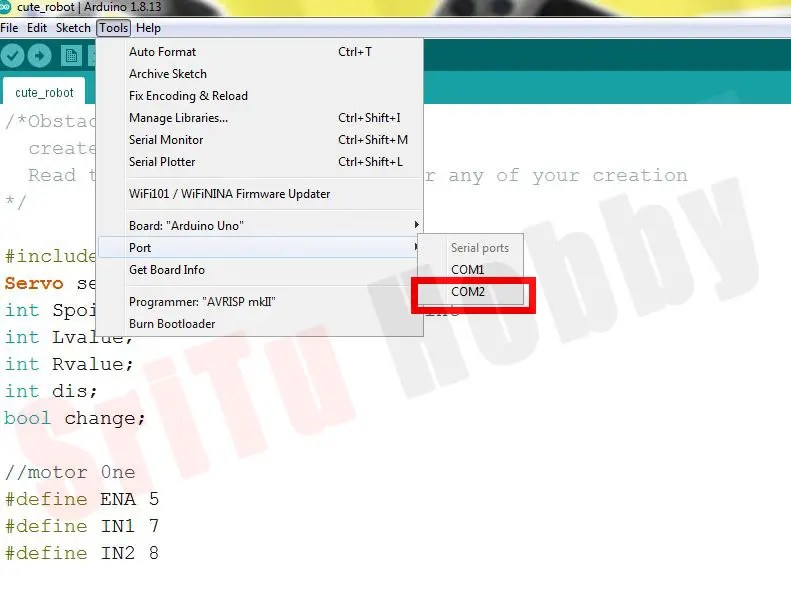### Step 13

Lastly, connect the power source, for which I use two cell phone batteries. You can use any other power source. But, keep in mind that you need to give the Arduino board a 7v to 12v VDC.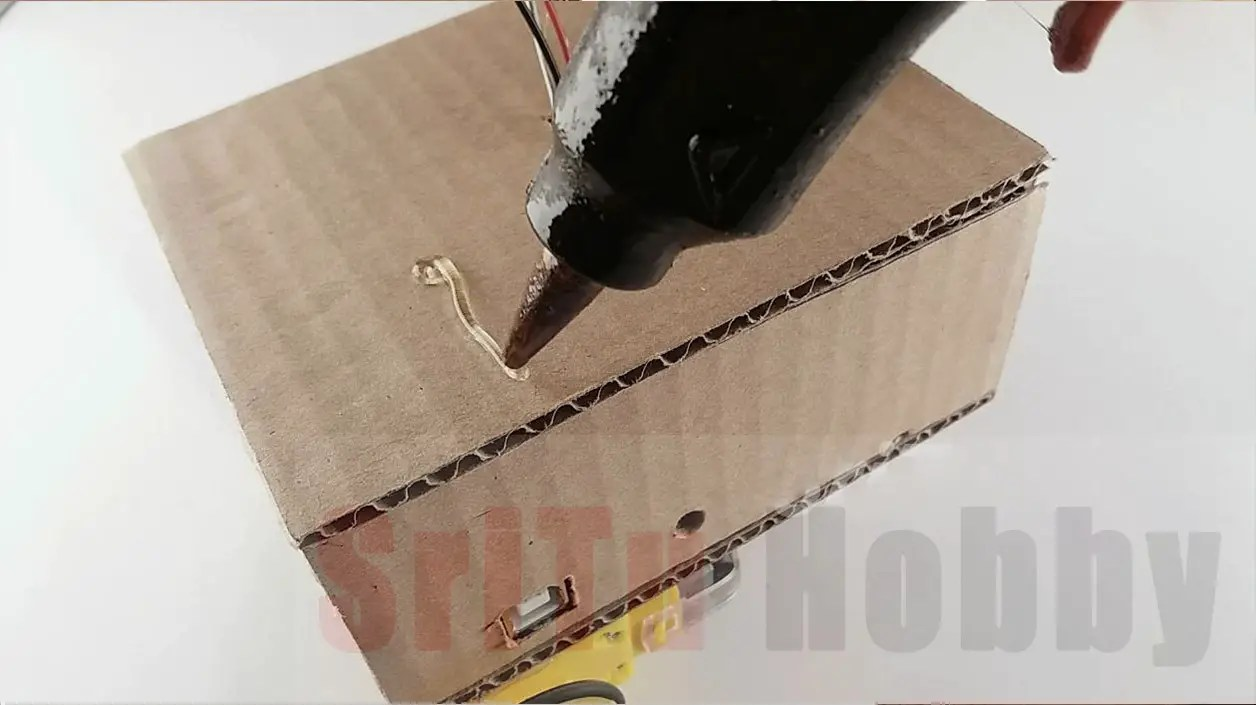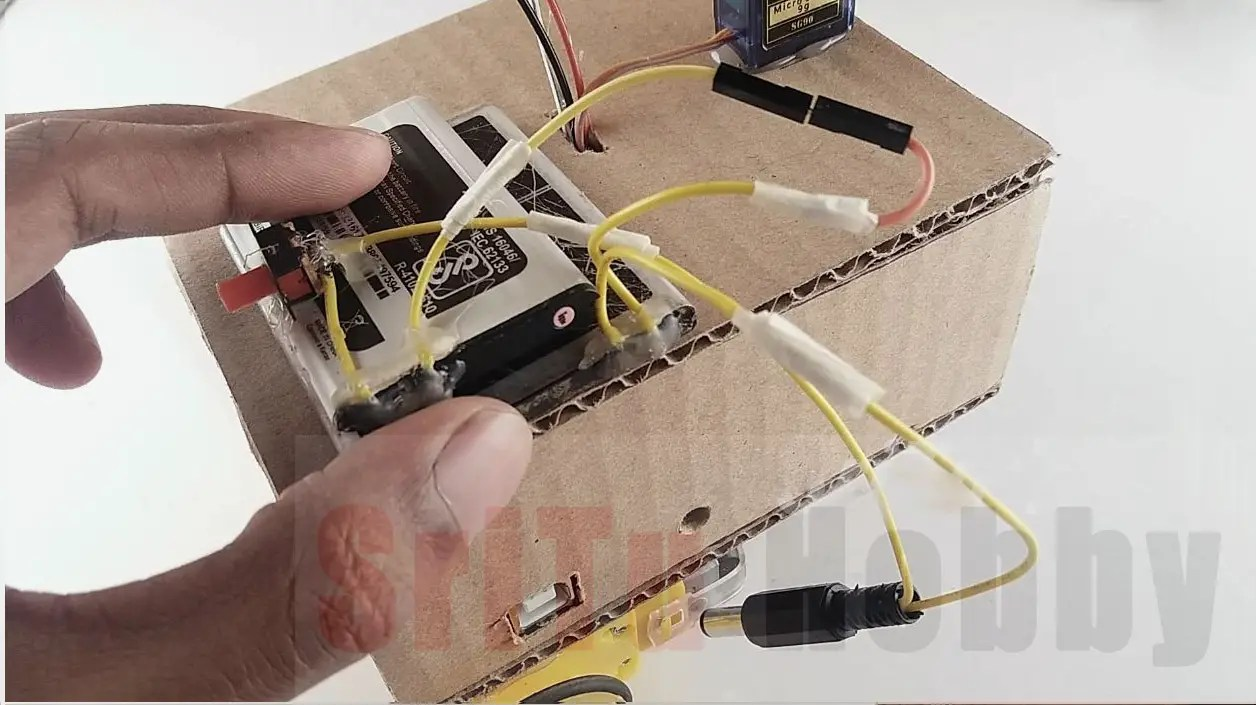OK, now enjoy this Arduino robot project. The full video guide is given below. We will meet in the next tutorial.